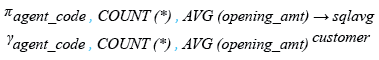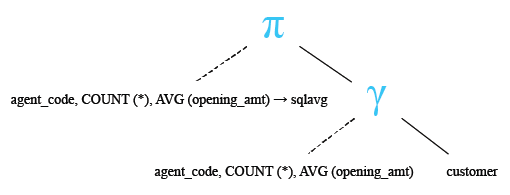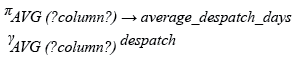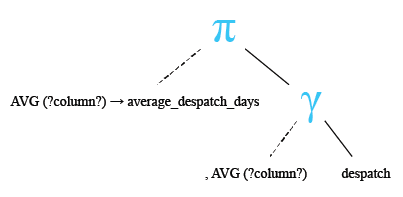# SQL CAST() inside AVG() for decimal value

## CAST() function inside AVG() function

The SQL AVG() function returns the average value with default decimal places. The CAST() is used to increase or decrease the decimal places of a value. The CAST() function is much better at preserving the decimal places when converting decimal and numeric data types. The 'AS DECIMAL' followed by the format specification is used with CAST() for making a numeric value to a specific decimal place value.

Syntax :

```CAST [ expression]
AS [data_type] [specified_format];
```

Parameters:

Name Description
expression Expression made up of a single constant, variable, scalar function, or column name and can also be the pieces of a SQL query that compare values against other values or perform arithmetic calculations.
data_type CHAR(), VARCHAR(), DECIMAL(), FLOAT etc.
specified_format Specified format.

Example :

To get the data the average of 'advance_amount' up to 2 decimal places form the 'orders' table, the following SQL statement can be used:

``````SELECT CAST(AVG(advance_amount) AS DECIMAL(10,2))
FROM orders;``````

Sample table: orders

Output:

```CAST(AVG(ADVANCE_AMOUNT)ASDECIMAL(10,2))
----------------------------------------
629.17
```

## SQL AVG() using CAST() inside the AVG()

To get the data of 'agent_code', number of customer, average of 'opening_amt' rounded upto two decimal with a heading 'SQLAVG' for each agent from the customer table with the following condition -

1. each 'agent_code' should come in a group

the following SQL statement can be used :

``````SELECT agent_code, COUNT(*),
AVG(CAST(opening_amt AS DECIMAL(12,2))) AS SQLAVG
FROM customer
ff GROUP BY agent_code;``````

Sample table: customer

Output:

```AGENT_CODE   COUNT(*)     SQLAVG
---------- ---------- ----------
A002                3 7333.33333
A004                3 8333.33333
A007                2       8000
A009                1       6000
A011                1       5000
A012                1       5000
A010                3 7333.33333
A001                1       8000
A008                3 4333.33333
A006                2       4000
A005                3 6333.33333
A003                2       6000
```

## SQL AVG() using CAST() outside the AVG()

To get the data of 'agent_code', number of customer, average of 'opening_amt' rounded upto two decimal with a heading 'SQLAVG' for each agent from the customer table with following condition -

1. each 'agent_code' should come in a group,

the following SQL statement can be used :

``````SELECT agent_code, COUNT(*),
CAST(AVG (opening_amt) AS DECIMAL (12,2)) AS SQLAVG
FROM customer
GROUP BY agent_code;``````

Sample table : customer

Output:

```AGENT_CODE   COUNT(*)     SQLAVG
---------- ---------- ----------
A002                3    7333.33
A004                3    8333.33
A007                2       8000
A009                1       6000
A011                1       5000
A012                1       5000
A010                3    7333.33
A001                1       8000
A008                3    4333.33
A006                2       4000
A005                3    6333.33
A003                2       6000
```

## SQL AVG() with COUNT()

To get the data of 'agent_code', number of each agent and average of 'opening_amt' for each agent with an user defined heading 'SQLAVG' from the customer table with following conditions -

1. each agent must be in a group,

2. and average should come with a heading 'SQLAVG',

the following SQL statement can be used:

``````SELECT agent_code, COUNT( * ),
AVG( opening_amt ) AS SQLAVG
FROM customer
GROUP BY agent_code;``````

Sample table: customer

Relational Algebra Expression:Relational Algebra Tree:Output:

```AGENT_CODE   COUNT(*)     SQLAVG
---------- ---------- ----------
A002                3 7333.33333
A004                3 8333.33333
A007                2       8000
A009                1       6000
A011                1       5000
A012                1       5000
A010                3 7333.33333
A001                1       8000
A008                3 4333.33333
A006                2       4000
A005                3 6333.33333
A003                2       6000
```

## SQL AVG() on datetime

To get the average of ('des_date' - 'ord_date') from the 'despatch' table, the following SQL statement can be used :

``````SELECT AVG (des_date - ord_date) AS average_despatch_days
FROM despatch;``````

Sample table: despatch

Relational Algebra Expression:Relational Algebra Tree:Output:

```AVERAGE_DESPATCH_DAYS
---------------------
-11
```

Note: Outputs of the said SQL statement shown here is taken by using Oracle Database 10g Express Edition

Here is a slide presentation of all aggregate functions.

Practice SQL Exercises

Previous: Avg function
Next: Avg with round, group by

﻿

## SQL: Tips of the Day

SQLite database default time value 'now':

```CREATE TABLE test (
id INTEGER PRIMARY KEY AUTOINCREMENT,
t TIMESTAMP
DEFAULT CURRENT_TIMESTAMP
);
```

Ref: https://bit.ly/3PC4Wsg